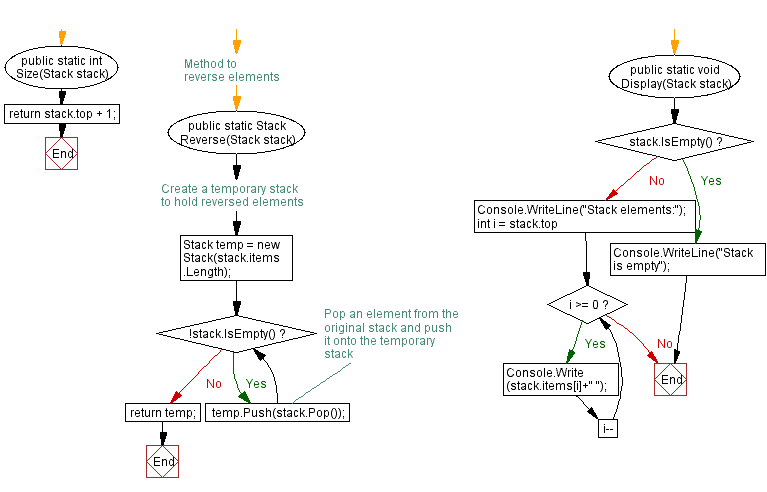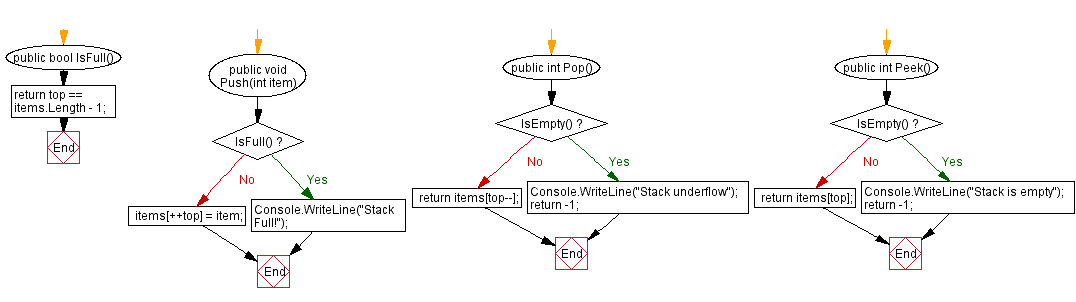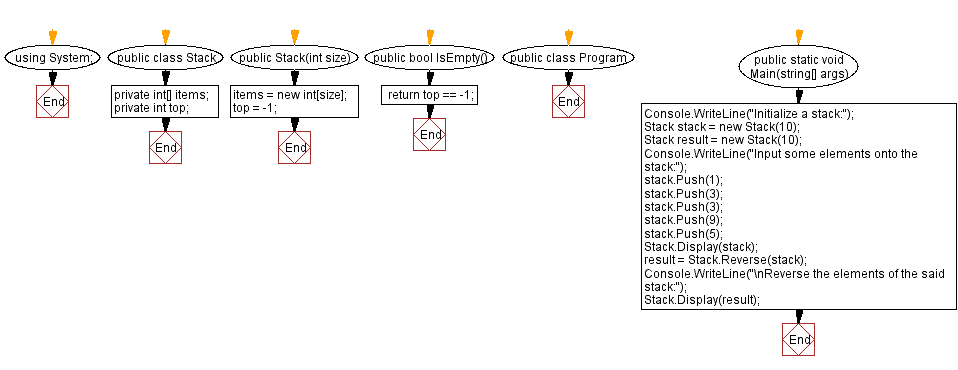﻿ C# - Reverse the elements of a stack

# C# Sharp Stack Exercises: Reverse the elements of a stack

## C# Sharp Stack: Exercise-4 with Solution

Write a C# program to reverse the elements of a given stack.

Sample Solution:

C# Code:

``````using System;
public class Stack
{
private int[] items;
private int top;

public Stack(int size)
{
items = new int[size];
top = -1;
}

public bool IsEmpty()
{
}

public bool IsFull()
{
}

public void Push(int item)
{
if (IsFull())
{
Console.WriteLine("Stack Full!");
return;
}

items[++top] = item;
}

public int Pop()
{
if (IsEmpty())
{
Console.WriteLine("Stack underflow");
return -1;
}

return items[top--];
}

public int Peek()
{
if (IsEmpty())
{
Console.WriteLine("Stack is empty");
return -1;
}

return items[top];
}

public static int Size(Stack stack)
{
return stack.top + 1;
}

// Method to reverse elements
public static Stack Reverse(Stack stack)
{
// Create a temporary stack to hold reversed elements
Stack temp = new Stack(stack.items.Length);

while (!stack.IsEmpty())
{
// Pop an element from the original stack and push it onto the temporary stack
temp.Push(stack.Pop());
}

return temp;

}

public static void Display(Stack stack)
{
if (stack.IsEmpty())
{
Console.WriteLine("Stack is empty");
return;
}

Console.WriteLine("Stack elements:");
for (int i = stack.top; i >= 0; i--)
{
Console.Write(stack.items[i]+" ");
}
}

}

public class Program
{
public static void Main(string[] args)
{
Console.WriteLine("Initialize a stack:");
Stack stack = new Stack(10);
Stack result = new Stack(10);
Console.WriteLine("Input some elements onto the stack:");
stack.Push(1);
stack.Push(3);
stack.Push(3);
stack.Push(9);
stack.Push(5);
Stack.Display(stack);
result = Stack.Reverse(stack);
Console.WriteLine("\nReverse the elements of the said stack:");
Stack.Display(result);
}
}
``````

Sample Output:

```Initialize a stack:
Input some elements onto the stack:
Stack elements:
5 9 3 3 1
Reverse the elements of the said stack:
Stack elements:
1 3 3 9 5
```

Flowchart:C# Sharp Code Editor:

Improve this sample solution and post your code through Disqus

What is the difficulty level of this exercise?

Test your Programming skills with w3resource's quiz.

﻿